×#### Thank you for registering.

One of our academic counsellors will contact you within 1 working day.

Click to Chat

1800-1023-196

+91-120-4616500

CART 0

• 0

MY CART (5)

Use Coupon: CART20 and get 20% off on all online Study Material

ITEM
DETAILS
MRP
DISCOUNT
FINAL PRICE
Total Price: Rs.

There are no items in this cart.
Continue Shopping• Complete JEE Main/Advanced Course and Test Series
• OFFERED PRICE: Rs. 15,900
• View Details

```Chapter 33: Probability – Exercise 33.4

Probability – Exercise 33.4 – Q.1(a)

Given,

P(A) = 0.4

P(B) = 0.5

∴ A and B are mutually exclusive events, then P(A ∩ B) = 0

Now,

(i) P(A ∩ B) = P(A) + P(B)

= 0.4 + 0.5

= 0.9

∴ P(A ∪ B) = 0.9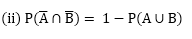= 1 - 0.9

= 0.1= 0.5 - 0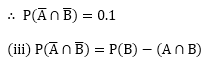= 0.4 - 0

= 0.4Probability – Exercise 33.4 – Q.1(b)

Given,

P(A) = 0.54

P(B) = 0.69

P(A ∩ B) = 0.35

(i) P(A ∪ B) = P(A) + P(B) - P(A ∩ B)

= 0.54 + 0.69 - 0.35

= 1.23 - 0.35

∴ P(A ∪ B) = 0.88= 1 - 0.88

= 0.12= 0.54 - 0.35

= 0.19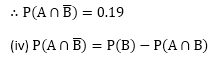= 0.69 - 0.35

= 0.34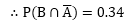Probability – Exercise 33.4 – Q.1(c)

(i) Given,(ii) Given,

P(A) = 0.35,  P(B) = ...

P(A ∩ B) = 0.25, P(A ∪ B) = 0.6

∵ P(A ∪ B) = P(A) + P(B) - P(A ∩ B)

0.6 = 0.35 + P(B) - 0.25

0.6 = 0.10 + P(B)

P(B) = 0.6 - 0.1

P(B) = 0.5

(iii) Given,

P(A) = 0.5,  P(B) = 0.35

P(A ∩ B) =....,  P(A ∪ B) = 0.7

∵ P(A ∪ B) = P(A) + P(B) - P(A ∩ B)

0.7 = 0.5 + 0.35 - P(A ∩ B)

0.7 = 0.85 - P(A ∩ B)

P(A ∩ B) = 0.85 - 0.7

P(A ∩ B) = 0.15

Probability – Exercise 33.4 – Q.2

We know by addition theorem on probability

P(A ∪ B) = P(A) + P(B) - P(A ∩ B)

⟹ 0.5 = 0.3 + 0.4 - P(A ∩ B)

P(A ∩ B) = 0.3 + 0.4 - 0.5

= 0.7 - 0.5

= 0.2

∴ P(A ∩ B) = 0.2

Probability – Exercise 33.4 – Q.3

We know by addition theorem on probability

P(A ∪ B) = P(A) + P(B) - P(A ∩ B)

= 0.5 + 0.3 - 0.2

= 0.8 - 0.2

= 0.6

∴ P(A ∪ B) = 0.6

Probability – Exercise 33.4 – Q.4

We know,

P(A ∪ B) = 0.8

P(A ∩ B) = 0.3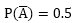⟹ 1 - P(A) = 0.5

⟹ P(A) = 1 - 0.5 = 0.5

Now, by addition theorem on probabiltiy

P(A ∪ B) = P(A) + P(B) - P(A ∩ B)

0.8 = 0.5 + P(B) - 0.3

0.8 = P(B) + 0.2

P(B) = 0.8 - 0.2

= 0.6

∴ P(B) = 0.6

Probability – Exercise 33.4 – Q.5

Given,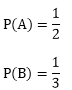∴ A and B are mutually exclusive events, then P(A ∩ B) = 0

P(A ∪ B) = P(A) + P(B)Probability – Exercise 33.4 – Q.6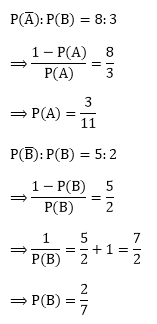∵ A, B and C are mutually exhaustive

∴  A ∪ B ∪ C = S
⟹ P(A ∪ B ∪ C) = P(S)

⟹ P(A) + P(B) + P(C) = 1

P(C) = 1 – {P(A) + P(B)}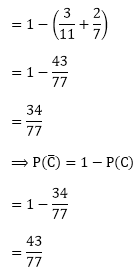∴ odds against C is= 43 : 34

Probability – Exercise 33.4 – Q.7

Let chance in favour of other be xOdds in favour of otherProbability – Exercise 33.4 – Q.8

∵ I card is drawn from a well shuffled deck of 52 cards

∴ S = 52C1 = 52

Now,

The favourable events is that drawn card is either spade or a king

Let A = Event of choosing shade

⟹ 13C1 = 13

B = Event of choosing a king

⟹ 4C1 = 4

Also, king can be of spade

∴ (A ∩ B) = 1

∴ P(A ∩ B) = P(A) + P(B) - P(A ∩ B)Probability – Exercise 33.4 – Q.9

Since two dice is thrown,

∴ S = 62 = 36

Let A be the event of choosing doublet

= {(1, 1), (2, 2), (3, 3), (4, 4), (5, 5), (6, 6)}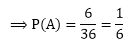B the event of choosing total of 9.

{(3, 6), (4, 5), (5, 4), (6, 3)}∴ Probability of choosing neither a doublet nor a total of 9.Now,

P(A ∪ B) = P(A) + P(B) - P(A ∩ B)Now,```### Course Features

• 728 Video Lectures
• Revision Notes
• Previous Year Papers
• Mind Map
• Study Planner
• NCERT Solutions
• Discussion Forum
• Test paper with Video Solution